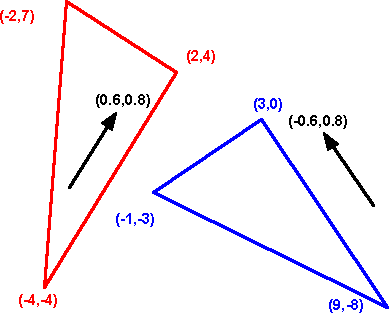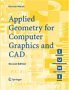# Maths - Intersection of 2D shapes

## Line intersectionThe intersection of two lines, if they do intersect, is a point.

If we have lines of infinite length then they will intersect unless the lines are parallel.

What is the best way to specify a line? In this case we want to work with finite length so a good way to specify the line is by its end points. In order to find the intersection of lines we need to convert this to the equation of the line: y = a*x + b as follows:

If the endpoints are: P1 and P2, then,

P1.y = a * P1.x + b
P2.y = a * P2.x + b

substituting for b gives:

P1.y - a * P1.x = P2.y - a * P2.x

a * (P2.x - P1.x) = (P2.y - P1.y)

therefore:

a = (P2.y - P1.y)/(P2.x - P1.x)

b = (P2.x*P1.y - P1.x*P2.y)/(P2.x - P1.x)

similarly, if we have a second line from P3 to P4 given by the equation y = c*x + d,

then we can find the intersection by solving the simultaneous equations:

y = a*x + b
y = c*x + d

substituting for y gives:

a*x + b = c*x + d

x * (a - c) = (d - b)

therefore the point of overlap is:

x = (d - b)/(a - c)

y = (a*d- b*c) /(a - c)

where:

• a = (P2.y - P1.y)/(P2.x - P1.x)
• b = (P2.x*P1.y - P1.x*P2.y)/(P2.x - P1.x)
• c = (P4.y - P3.y)/(P4.x - P3.x)
• d = (P4.x*P3.y - P3.x*P4.y)/(P4.x - P3.x)

These lines overlap if this point is between P1 and P2 and also between P3 and P4.

## Bounding Circles

The shape of overlapping circles is quite complicated and I'm not sure there is an easier way to specify the shape of intersecting circles than by specifying the two circles.

This is the easiest way to detect collisions because a circle is independent of alignment.

Two circles overlap if the sum of there radii is greater than the distance between their centers. Therefore by Pythagoras we have a collision if:

(cx1-cx2)2 + (cy1-cy2)2 < (r1+r2)2

where:

• cx1,cy1 = centre x and y coordinates of circle 1
• cx2,cy2 = centre x and y coordinates of circle 2
• r1 = radius of circle 1
• r2 = radius of circle 2## Triangles

The intersection of two triangles could be a 3 to 6 sided polygon.

In order to check if the triangles do overlap we need to look round the triangles to see if there is clear space between the two triangles. In order to do that, in a way that can be done by a computer, we project all the points on both triangles onto a line. If we can find a line which has the points of one triangle and one end and the points of the other triangle at the other end then we have found a gap between the two triangles and they don't overlap.

How do we find a line orientation that will find the gap (if it exists) between the two triangles? Well we could try all possible orientations from 0 to 360 degrees but we don't have to do this. If we try the 6 orientations perpendicular to the sides of both triangles that should do it.## Bounding Rectangles

### axis aligned

How do we detect when these rectangles overlap? If the axis of the rectangles are aligned it is easy to check if they overlapped

### non-axis aligned

.If we were checking for a collision in the physical world we could walk around the objects, if we could see could see a gap between the objects from any direction, then the objects cant be overlapping.Since the objects are rectangular we only need to check along the planes of the rectangles which gives us four planes to check. We project the corner points of each rectangle onto each line. If the points of one rectangle is at one end of the line and the other rectangle is at the other end, with a gap in between, then the objects have not collided. We only need to find one line without an overlap to be sure there has not been a collision, only if all four lines have overlaps has a collision occurred.

In the diagram above, the line on the bottom left does not have an overlap.

Projecting onto a line is explained on this page. Since we only need to know how far along the vector the projected point is, all we need to do is take the dot product of the vector and the point, the result of this is a scalar quantity which is what we want.

To rotate the vector through 90 degrees we just swap the x and y coordinates.

## Example

In this example we have two cars, the orientation of the red one is (0.6,0.8) and the orientation of the blue one is (-0.6,0.8).

Lets first first project all the points onto the four possible vectors (here is the spreadsheet I used to calculate it collision.ods)

 dot red dot red 90 degrees dot blue dot blue 90 degrees red vector 0.6 0.8 blue vector -0.6 0.8 red -2 7 4.4 2.6 6.8 -5.8 red 2 4 4.4 4 2 -0.8 red -4 -4 -5.6 -5.6 -0.8 -0.8 red -8 -1 -5.6 -7 4 -5.8 blue 3 0 1.8 2.4 -1.8 2.4 blue 9 -8 -1 2.4 -11.8 12 blue 5 -11 -5.8 -2.6 -11.8 10.6 blue -1 -3 -3 -2.6 -1.8 1

As you can see the column marked 'dot blue' does not overlap (red points are greater than -1, blue points are less than -1). So the cars do not overlap. The 4th column does not overlap either, but we only need to find one to reject the possibility of a collision.

 metadata block see also: Correspondence about this page Book Shop - Further reading. Where I can, I have put links to Amazon for books that are relevant to the subject, click on the appropriate country flag to get more details of the book or to buy it from them.Applied Geometry for Computer Graphics...

This site may have errors. Don't use for critical systems.